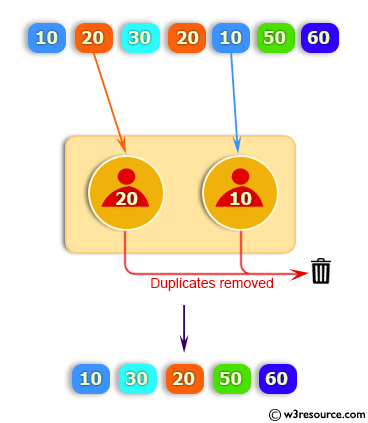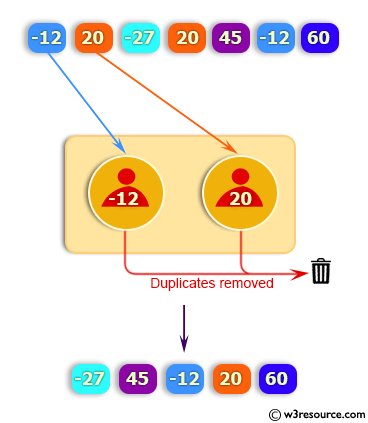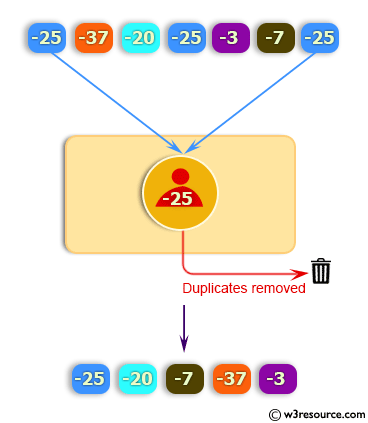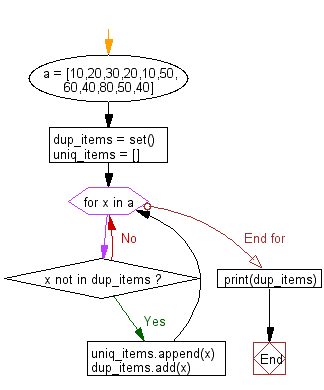﻿ Python: Remove duplicates from a list - w3resource# Python: Remove duplicates from a list

## Python List: Exercise-7 with Solution

Write a Python program to remove duplicates from a list.

Example - 1 :Example - 2 :Example - 3 :Sample Solution:-

Python Code:

``````a = [10,20,30,20,10,50,60,40,80,50,40]

dup_items = set()
uniq_items = []
for x in a:
if x not in dup_items:
uniq_items.append(x)

print(dup_items)
```
```

Sample Output:

```{40, 10, 80, 50, 20, 60, 30}
```

Flowchart:## Visualize Python code execution:

The following tool visualize what the computer is doing step-by-step as it executes the said program:

Python Code Editor:

Have another way to solve this solution? Contribute your code (and comments) through Disqus.

What is the difficulty level of this exercise?

Test your Python skills with w3resource's quiz

﻿

## Python: Tips of the Day

Floor Division:

When we speak of division we normally mean (/) float division operator, this will give a precise result in float format with decimals.

For a rounded integer result there is (//) floor division operator in Python. Floor division will only give integer results that are round numbers.

```print(1000 // 300)
print(1000 / 300)```

Output:

```3
3.3333333333333335```